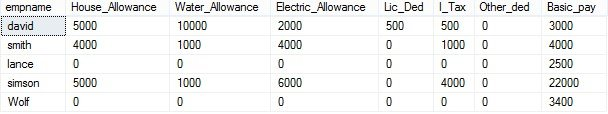DatabaseLearn different types of operators in SQL

# Learn different types of operators in SQL

In this article we will learn different types of operators in SQL. These operators are used with SQL select statement or SQL clause to get the required output.

What is an operator?

• An operator is used to limit the number of row in result set.
• Commonly used SQL operator are given below:
1. Arithmetic Operator
2. Comparison Operator
3. Logical Operator

Let’s discuss the use of each operator in detail:

1. Arithmetic Operator:
• Arithmetic operator used to perform mathematical operations.
• It must be used with numbers (integer, float and double)
• Let’s have a look at the following salary table :
• On this salary table, we are going to perform addition operation and subtraction operation for calculating a salary.

Basic formula for calculating salary:

`(Basic pay + Allowances)   - (Deduction)`

Query:

`select empname, (House_Allowance + Water_Allowance + Electric_Allowance + Basic_pay)  - (Lic_Ded+I_Tax+Other_ded) as salary from salary  `
• Similarly, you can perform other arithmetic operations with the available arithmetic operator’s to manipulate the result set.

2. Comparison Operator:
• These operators are used to compare data in column to the data specified in SQL statement.
• The comparison operators should be used with where clause.
• It limits the result set specified by particular comparison operator.
• Let’s understand the uses of these operators in the following example, where we will use one of the comparison operator to manipulate the data in salary table:Query: To get the employee whose basic pay is more than 3000

`select  empname,Basic_pay from salary where Basic_pay >3000`
3. Logical Operator:
• AND, OR, NOT are the three types of logical operators.
• It populate’s the result only If the condition is true.
• It result’s into boolean data type, if condition is true then it populates the result set like comparison operator.
• Let see the implementation of one of the logical operator with salary table:Query: To get salary of employee whose basic pay is between 2000 to 5000 using AND operator.

`select  empname,Basic_pay from salary where Basic_pay >=2000 and Basic_pay <=5000`
• Similarly, you can implement other logical operators to manipulate the data.

Hence, we successfully learnt about different types of operators that are used in SQL along with their implementations.

### Why Is Design Thinking Important In Digital Transformation?

21,501Fans
4,210Followers
104,000Subscribers

### Why Is Design Thinking Important In Digital Transformation?

- Advertisement -